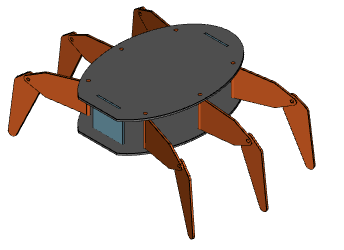# rotate and translate assemblies

I followed “Is there a vtkPropAssembly class we can use in vtkjs? · Issue #1319 · Kitware/vtk-js · GitHub” and created an vtkAssembly class with `getNestedProps`, `getBounds` and `getActors` (see below). The visualisation works nicely.

Now I’d like to rotate a subassembly, e.g. rotating the left front leg of a hexapod around the rotation axis of the upper leg:Applying `rotateZ` on the vtkAssembly doesn’t do anything. I can only apply it to the included `vtkActor`s. However, I want to apply the rotation of the lower leg also around the rotation axis of the upper leg and not of the lower leg (I think that is what assemblies are meant for). What do I need to implement additionally in my vtkAssembly class? Do I need to create hierarchical versions of `rotateZ` and all the other functions? And how would I propagate the same rotation down the hierarchy?

Any hint would be appreciated!

``````import macro from '@kitware/vtk.js/macro'
import vtkProp3D from '@kitware/vtk.js/Rendering/Core/Prop3D';

function vtkAssembly(publicAPI, model) {
model.classHierarchy.push('vtkAssembly');
model.actors = [];
model.bounds = null;

publicAPI.getNestedProps = () => {
return model.actors;
};

publicAPI.addActor = (actor) => {
model.actors.push(actor);
};

publicAPI.getActors = () => {
var actors = [];
model.actors.forEach(actor => {
if (actor.getClassName() == "vtkAssembly") {
actors = [...actors, ...actor.getActors()];
} else {
actors.push(actor);
}
});
return actors
}

publicAPI.getBounds = () => {
if (model.bounds == null) {
var bounds = null;
model.actors.forEach(a => {
if (bounds == null) {
bounds = a.getBounds();
} else {
var bounds2 = a.getBounds();
for (var i = 0; i < 3; i++) {
bounds[2 * i] = Math.min(bounds[2 * i], bounds2[2 * i])
bounds[2 * i + 1] = Math.max(bounds[2 * i + 1], bounds2[2 * i + 1])
}
}
model.bounds = bounds;
});
}
return model.bounds
}
}

export function extend(publicAPI, model, initialValues = {}) {
Object.assign(model, initialValues);
vtkProp3D.extend(publicAPI, model, initialValues);
vtkAssembly(publicAPI, model);
}

// ----------------------------------------------------------------------------

export const newInstance = macro.newInstance(extend, 'vtkAssembly');

// ----------------------------------------------------------------------------

export default { newInstance, extend };``````
1 Like